# E-PolyLearning

 1. The following question consists of two sets of figures. Figures A, B, C and D constitute the Problem Set while figures 1, 2, 3, and 4 constitute the Answer Set. There is a definite relationship between figures A and B. Establish a similar relationship between figures C and D by selecting a suitable figure from the Answer Set that would replace the question mark (?) in fig. (D).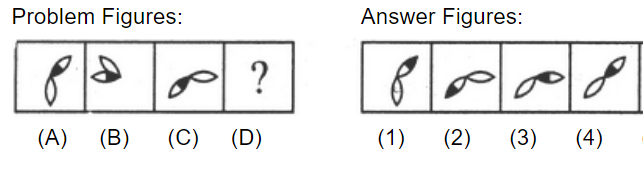a. 1 b. 2 c. 3 d. 4

 2. The following question consists of two sets of figures. Figures A, B, C and D constitute the Problem Set while figures 1, 2, 3, and 4 constitute the Answer Set. There is a definite relationship between figures A and B. Establish a similar relationship between figures C and D by selecting a suitable figure from the Answer Set that would replace the question mark (?) in fig. (D).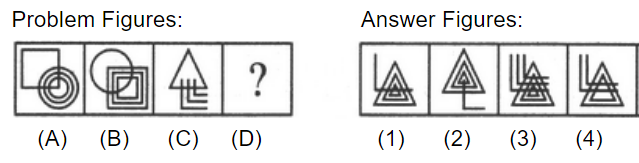a. 1 b. 2 c. 3 d. 4
 3. The following question consists of two sets of figures. Figures A, B, C and D constitute the Problem Set while figures 1, 2, 3, and 4 constitute the Answer Set. There is a definite relationship between figures A and B. Establish a similar relationship between figures C and D by selecting a suitable figure from the Answer Set that would replace the question mark (?) in fig. (D).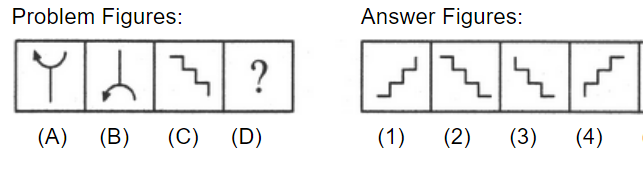a. 1 b. 2 c. 3 d. 4
 4. The following question consists of two sets of figures. Figures A, B, C and D constitute the Problem Set while figures 1, 2, 3, and 4 constitute the Answer Set. There is a definite relationship between figures A and B. Establish a similar relationship between figures C and D by selecting a suitable figure from the Answer Set that would replace the question mark (?) in fig. (D).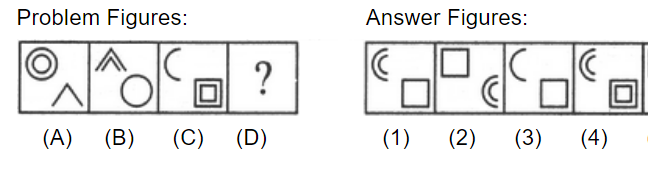a. 1 b. 2 c. 3 d. 4
 5. The following question consists of two sets of figures. Figures A, B, C and D constitute the Problem Set while figures 1, 2, 3, and 4 constitute the Answer Set. There is a definite relationship between figures A and B. Establish a similar relationship between figures C and D by selecting a suitable figure from the Answer Set that would replace the question mark (?) in fig. (D).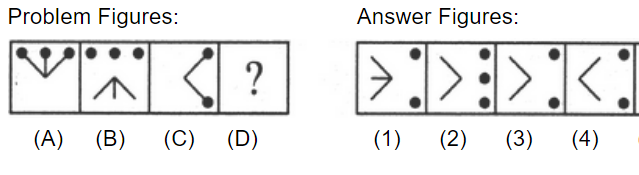a. 1 b. 2 c. 3 d. 4